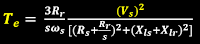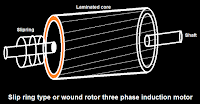# speed control methods of three phase induction motor?

speed control methods of three phase induction motor?

Speed control of three phase induction motor

•There are two types of AC motor one is synchronous motor and second is asynchronous motor (induction motor).

•Three phase induction motor is a self starting motor.

•Motor have two main parts one is stator and second is rotor.

•Speed of three phase induction motor can be control from stator side and rotor side.

•The main method for controlling speed of induction motor are one is by changing number of poles second is by stator voltage control third is by V/F control and fourth is by rotor resistance control.

By changing number of poles :

•This method is use for squirrel cage rotor motor only.•The number of stator poles can be change by three methods one is multiple stator winding and second is method of consequent poles and third is pole amplitude modulation.

•We have,"    N_s =  120f/P

•Where, N_s  is the synchronous speed , f is the frequency , P is the number of poles.

•So by changing the number of poles synchronous speed changes.

By stator voltage control :

•We have,•Torque is directly proportional to stator voltage.

•This method is very easy and cheap but still use rarely.

•Because by decreasing the voltage torque will reduce.

•But this will increase current in motor which will heat the motor which is not good it causes losses.

•Also the torque is proportional to square of voltage so large voltage change is required for changing torque.

By V/F control :

•We have,  N_s=  120f/P

•Where, N_s  is the synchronous speed , f is the frequency , P is the number of poles.

•Also, V=4.44φfTK  ( which means φ α V/f ).

•Where, V is the voltage , φ is the flux , f is the frequency , T is number of turns per phase , K is the winding constant.

•If we increase the frequency (f) flux (φ) will decrease because they are inversely proportional and if we increase the voltage (V ) flux (φ) will increase because they are directly proportional.

•In this method we have to keep V/f ratio constant.

By Rotor resistance control :

•We have, Torque α slip/(rotor resistance)

•This method is use for wound rotor motor only.•By adding external variable resistance to rotor via slip ring we can control the speed of motor.

•It can also use as starter.

•It is simple method.

•But there is resistance means heat means losses means less efficiency.

← Prev Question Next Question →